# Convolutional Filter Factorization¶

In this notebook, I want to see if we can factorize a spatial filter with large support into successive convolutions of filters with smaller spatial support. The inspiration for this comes from the fact that I often have to work with large spatial kernels, and there may be some speedup available by using successive smaller convolutions.

For example lots of 5x5 kernels can be made up of 2 3x3 convolutions, using 18 multiply/adds per pixel instead of 25. Of course, there are some that cannot be made up from 2 3x3 convolutions, but perhaps we are happy to pay a small error term.

Further, when viewing nvidia-prof profiling outputs, it seems that if we use 3x3 convolutions, these are done using winograd rather than fft/ifft lowlevel kernels. I wouldn’t be surprised if due to the large prevalence of 3x3 kernels in the machine learning literature, that GPUs are being specifically optimized for them.

In :

# Import my plotting libraries. plotters is available at
# https://github.com/fbcotter/plotters
%matplotlib notebook
import matplotlib as mpl
mpl.rcParams['image.resample'] = False
mpl.rcParams['image.interpolation'] = 'none'
mpl.rcParams['image.cmap'] = 'gray'
import matplotlib.pyplot as plt
import plotters

# Import my numeric libraries. Pytorch wavelets is a library that allows
# the dtcwt kernels (the particular ones I want to speed up) to be done in
# pytorch (and optionally, with a GPU).
import numpy as np
import torch
import torch.nn.functional as F
import dtcwt
from pytorch_wavelets import DTCWTForward, DTCWTInverse
from pytorch_wavelets.dtcwt.lowlevel import q2c
dev = torch.device('cpu')


# Get the target filters¶

This next bit of code is largely specific to my task. Any filters here would suffice. For our example, I’m looking at the synthesis filters of a single layer inverse Dual Tree Complex Wavelet Transform. They are 7x7 but have little energy in the outermost pixels.

In :

ifm = DTCWTInverse(J=1, biort='near_sym_a', qshift='qshift_a')

def yb_to_yh(yb):
# Converts the 6 complex bands back into the 3 LH, HH, HL bands
deg15, deg165 = q2c(yb[:,0])
deg45, deg135 = q2c(yb[:,1])
deg75, deg105 = q2c(yb[:,2])
yh = torch.stack((deg15, deg45, deg75, deg105, deg135, deg165), dim=2)
return yh

# Create an output array to store the results
xb = np.zeros((4,16,16))
yl = torch.zeros(1,1,16,16)
yb = torch.zeros(1,3,1,16,16)

# Put impulses in the ll, lh, hh and hl bands
yl[0,0,8,8] = 1
x = ifm((yl, [yb_to_yh(yb)]))
xb = x.detach().cpu().numpy()[0,0]
yl[0,0,8,8] = 0
for b in range(3):
yb[0,b,0,8,8] = 1
x = ifm((yl, [yb_to_yh(yb)]))
xb[b+1] = x.detach().cpu().numpy()[0,0]
yb[0,b] = 0

# Plot the resulting outputs
fig, ax = plt.subplots(1,4, figsize=(9,3),
subplot_kw={'xticks':[], 'yticks':[]},
gridspec_kw={'top':0.95, 'bottom':0.05, 'left':0.05,
'right':0.95, 'wspace':0.05})
for i in range(4):
ax[i].imshow(xb[i])
fig.suptitle('ll, lh, hh, hl impulse responses - our 7x7 target filters');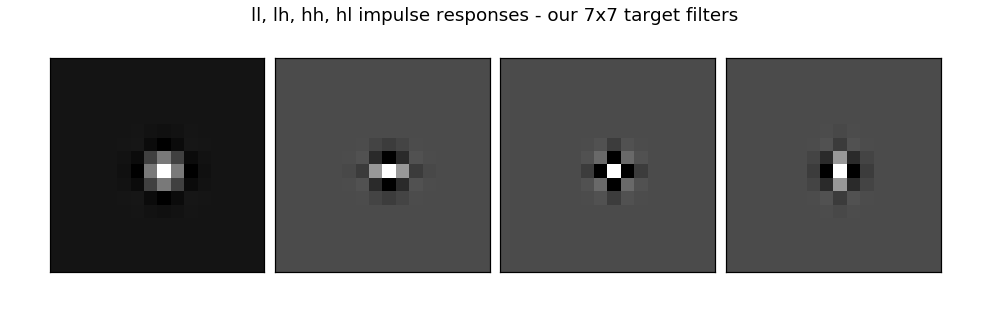# Aside 1: Convex vs Non Convex¶

This is a non convex optimization problem. Consider the simple case where we want to minimize a 1x1 kernel as a convolution of 2 1x1 kernels (I know this is silly, but it makes the maths nicer). Then our output is:

$f = xy$

To check for convexity, we need the Hessian matrix to be positive semi definite. We know that whenever there is a cross term then the problem cannot be convex, but for simplicity sake, let us check what the Hessian matrix is anyway.

$H_{i,j} = \frac{\partial^2f}{\partial x_i \partial x_j}$
$\begin{split}H = \begin{bmatrix} 0 & 1 \\ 1 & 0 \end{bmatrix}\end{split}$

Positive semi definite means that for any $$x$$, $$x^THx$$ must be greater than or equal to zero for any x. In our above problem, our Hessian is larger than 2 by 2, but there is a nice trick in that every subset of the Hessian must also be PSD (you can set the corresponding parts of $$x$$ to 0 to ignore the other areas). In the above case, if $$x=[-1,1]^T$$ gives $$x^THx = -2$$.

Another nice bit of info for PSD, is that if a matrix is diagonally dominant, i.e.

$h_{i,i} \geq \sum_{j \neq i} |h_{i,j}|$

and $$h_{i,i} \geq 0$$, then we have a PSD matrix. This however is sufficient and not necessary. This is often why we sometimes add a small identity matrix to a covariance matrix to make sure we are satisfying its PSD conditions.

# Aside 2: check how to do alternating projections¶

Non convex optimization is not trivial. A simple way of solving it is to do alternating projections. I.e. minimize w.r.t. x keeping y constant, the minimizing w.r.t. y keeping x constant. Let us return to the $$f=xy$$ problem, and try to use pytorch to alternate minimizations. This is an underdetermined set of equations, so let us also add a constraint on the norms of $$x$$ and $$y$$. I.e. minimize:

$L = (xy - \alpha)^2 + \lambda (|x| + |y|)$

As an example, let us try to find two numbers $$x$$ and $$y$$ that multiply to give 20, while minimising the $$l_1$$ norm.

In :

# Randomly initialize x and y to be a gaussian with std 10

# Set the target (α), the lagrangian multiplier (λ) and the learning rate
α = 20
λ = 0.1
lr = 1e-2
N = 20 # Number of steps

# Create two optimizers, one for x and one for y
optimx = torch.optim.SGD([x], lr=lr, momentum=0)
optimy = torch.optim.SGD([y], lr=lr, momentum=0)
for i in range(N):
# Minimize wrt x
z = x*y
l = (z - α)**2 + λ * (torch.abs(x) + torch.abs(y))
print("Step {:2}a: Loss = {loss:09.4f}\tOut = {out:.2f} ({x:.2f}, {y:.2f})".format(
i, loss=l.item(), out=z.item(), x=x.item(), y=y.item()))
l.backward()
optimx.step()

# Minimize wrt y
z = x*y
l = (z - α)**2 + λ * (torch.abs(x) + torch.abs(y))
print("Step {:2}b: Loss = {loss:09.4f}\tOut = {out:.2f} ({x:.2f}, {y:.2f})".format(
i, loss=l.item(), out=z.item(), x=x.item(), y=y.item()))
l.backward()
optimy.step()

Step  0a: Loss = 0404.3896      Out = -0.10 (0.04, -2.68)
Step  0b: Loss = 0296.7857      Out = 2.78 (-1.04, -2.68)
Step  1a: Loss = 0284.1869      Out = 3.15 (-1.04, -3.03)
Step  1b: Loss = 0189.4539      Out = 6.25 (-2.06, -3.03)
Step  2a: Loss = 0158.8276      Out = 7.42 (-2.06, -3.60)
Step  2b: Loss = 0087.5617      Out = 10.68 (-2.97, -3.60)
Step  3a: Loss = 0059.7771      Out = 12.31 (-2.97, -4.15)
Step  3b: Loss = 0026.1703      Out = 14.96 (-3.60, -4.15)
Step  4a: Loss = 0014.7589      Out = 16.27 (-3.60, -4.51)
Step  4b: Loss = 0005.7598      Out = 17.78 (-3.94, -4.51)
Step  5a: Loss = 0003.2123      Out = 18.47 (-3.94, -4.69)
Step  5b: Loss = 0001.6230      Out = 19.14 (-4.08, -4.69)
Step  6a: Loss = 0001.2203      Out = 19.42 (-4.08, -4.76)
Step  6b: Loss = 0000.9932      Out = 19.68 (-4.14, -4.76)
Step  7a: Loss = 0000.9386      Out = 19.78 (-4.14, -4.78)
Step  7b: Loss = 0000.9088      Out = 19.88 (-4.16, -4.78)
Step  8a: Loss = 0000.9019      Out = 19.92 (-4.16, -4.79)
Step  8b: Loss = 0000.8980      Out = 19.95 (-4.16, -4.79)
Step  9a: Loss = 0000.8972      Out = 19.96 (-4.16, -4.80)
Step  9b: Loss = 0000.8967      Out = 19.98 (-4.17, -4.80)
Step 10a: Loss = 0000.8966      Out = 19.98 (-4.17, -4.80)
Step 10b: Loss = 0000.8965      Out = 19.98 (-4.17, -4.80)
Step 11a: Loss = 0000.8965      Out = 19.99 (-4.17, -4.80)
Step 11b: Loss = 0000.8965      Out = 19.99 (-4.17, -4.80)
Step 12a: Loss = 0000.8965      Out = 19.99 (-4.17, -4.80)
Step 12b: Loss = 0000.8965      Out = 19.99 (-4.17, -4.80)
Step 13a: Loss = 0000.8965      Out = 19.99 (-4.17, -4.80)
Step 13b: Loss = 0000.8965      Out = 19.99 (-4.17, -4.80)
Step 14a: Loss = 0000.8965      Out = 19.99 (-4.17, -4.80)
Step 14b: Loss = 0000.8965      Out = 19.99 (-4.17, -4.80)
Step 15a: Loss = 0000.8965      Out = 19.99 (-4.17, -4.80)
Step 15b: Loss = 0000.8965      Out = 19.99 (-4.17, -4.80)
Step 16a: Loss = 0000.8965      Out = 19.99 (-4.17, -4.80)
Step 16b: Loss = 0000.8965      Out = 19.99 (-4.17, -4.80)
Step 17a: Loss = 0000.8965      Out = 19.99 (-4.17, -4.80)
Step 17b: Loss = 0000.8965      Out = 19.99 (-4.17, -4.80)
Step 18a: Loss = 0000.8965      Out = 19.99 (-4.17, -4.80)
Step 18b: Loss = 0000.8965      Out = 19.99 (-4.17, -4.80)
Step 19a: Loss = 0000.8965      Out = 19.99 (-4.17, -4.80)
Step 19b: Loss = 0000.8965      Out = 19.99 (-4.17, -4.80)


This seems to work nicely. Notice as we step from $$n$$a to $$n$$b, only one of the two parameters changes.

Now onwards to the filter factorization!

# Define filters as convolution of 2 3x3¶

Let us try match the lh, hl and hh wavelets. To make our filters admissable wavelets, we also need to impose the restriction that $$\sum_{i,j} w_{i,j} = 0$$

In :

# Define our

# Define the lagrangian for mse, minimum energy coefficients and admissability
λ = 100
λ1 = .1
λ2 = 1
lr = 5e-2
N = 20

y = torch.tensor(xb, dtype=torch.float32).reshape(1,1,16,16)
# Make it zero mean and unit std
y = (y-y.mean())/y.std()

# Define our two optimizers
optim1 = torch.optim.SGD([w1], lr=lr, momentum=0)
optim2 = torch.optim.SGD([w2], lr=lr, momentum=0)

x = torch.zeros(1,1,16,16)
x[0,0,8,8] = 1
lbest = np.inf
w1_best = w1.data.numpy()
w2_best = w2.data.numpy()

for i in range(N):
# Minimize wrt w1

lmse = λ * torch.mean((y - y2)**2)
lreg = λ1 * (torch.mean(w1**2) + torch.mean(w2**2))
l = lmse + lreg + ladmiss

print("Step {:2}a: Loss = {loss:09.4f}\tMSE: {mse:07.3f}\t "
i, loss=l.item(), mse=lmse.item(), reg=lreg.item(),
l.backward()
optim1.step()

if l.item() < lbest:
w1_best = w1.data.numpy()
w2_best = w2.data.numpy()
lbest = l.item()

# Minimize wrt w2

lmse = λ * torch.mean((y - y2)**2)
lreg = λ1 * (torch.mean(w1**2) + torch.mean(w2**2))
l = lmse + lreg + ladmiss

print("Step {:2}b: Loss = {loss:09.4f}\tMSE: {mse:07.3f}\t "
i, loss=l.item(), mse=lmse.item(), reg=lreg.item(),
l.backward()
optim2.step()

if l.item() < lbest:
w1_best = w1.data.numpy()
w2_best = w2.data.numpy()
lbest = l.item()

print('-' * 50)
print('Best loss was {}'.format(lbest))

Step  0a: Loss = 0104.7647      MSE: 103.354     Reg: 000.168   Admiss: 001.243)
Step  0b: Loss = 0043.8679      MSE: 043.400     Reg: 000.180   Admiss: 000.288)
Step  1a: Loss = 0022.3645      MSE: 021.798     Reg: 000.226   Admiss: 000.341)
Step  1b: Loss = 0009.5339      MSE: 008.788     Reg: 000.252   Admiss: 000.494)
Step  2a: Loss = 0006.9480      MSE: 006.337     Reg: 000.260   Admiss: 000.351)
Step  2b: Loss = 0003.7069      MSE: 003.242     Reg: 000.263   Admiss: 000.201)
Step  3a: Loss = 0002.5059      MSE: 002.113     Reg: 000.263   Admiss: 000.129)
Step  3b: Loss = 0001.6599      MSE: 001.254     Reg: 000.265   Admiss: 000.141)
Step  4a: Loss = 0001.2352      MSE: 000.890     Reg: 000.266   Admiss: 000.079)
Step  4b: Loss = 0000.9242      MSE: 000.644     Reg: 000.267   Admiss: 000.014)
Step  5a: Loss = 0000.7898      MSE: 000.515     Reg: 000.265   Admiss: 000.009)
Step  5b: Loss = 0000.7030      MSE: 000.419     Reg: 000.265   Admiss: 000.019)
Step  6a: Loss = 0000.6378      MSE: 000.362     Reg: 000.265   Admiss: 000.010)
Step  6b: Loss = 0000.5727      MSE: 000.305     Reg: 000.265   Admiss: 000.003)
Step  7a: Loss = 0000.5402      MSE: 000.274     Reg: 000.264   Admiss: 000.002)
Step  7b: Loss = 0000.5080      MSE: 000.243     Reg: 000.264   Admiss: 000.001)
Step  8a: Loss = 0000.4896      MSE: 000.225     Reg: 000.264   Admiss: 000.000)
Step  8b: Loss = 0000.4690      MSE: 000.204     Reg: 000.264   Admiss: 000.001)
Step  9a: Loss = 0000.4556      MSE: 000.192     Reg: 000.264   Admiss: 000.000)
Step  9b: Loss = 0000.4416      MSE: 000.178     Reg: 000.264   Admiss: 000.000)
Step 10a: Loss = 0000.4330      MSE: 000.169     Reg: 000.263   Admiss: 000.000)
Step 10b: Loss = 0000.4227      MSE: 000.159     Reg: 000.263   Admiss: 000.000)
Step 11a: Loss = 0000.4164      MSE: 000.153     Reg: 000.263   Admiss: 000.000)
Step 11b: Loss = 0000.4086      MSE: 000.145     Reg: 000.263   Admiss: 000.000)
Step 12a: Loss = 0000.4035      MSE: 000.140     Reg: 000.263   Admiss: 000.000)
Step 12b: Loss = 0000.3980      MSE: 000.135     Reg: 000.263   Admiss: 000.000)
Step 13a: Loss = 0000.3939      MSE: 000.131     Reg: 000.263   Admiss: 000.000)
Step 13b: Loss = 0000.3890      MSE: 000.126     Reg: 000.263   Admiss: 000.000)
Step 14a: Loss = 0000.3857      MSE: 000.123     Reg: 000.263   Admiss: 000.000)
Step 14b: Loss = 0000.3818      MSE: 000.119     Reg: 000.263   Admiss: 000.000)
Step 15a: Loss = 0000.3789      MSE: 000.116     Reg: 000.263   Admiss: 000.000)
Step 15b: Loss = 0000.3762      MSE: 000.113     Reg: 000.263   Admiss: 000.001)
Step 16a: Loss = 0000.3738      MSE: 000.111     Reg: 000.262   Admiss: 000.001)
Step 16b: Loss = 0000.3707      MSE: 000.108     Reg: 000.262   Admiss: 000.000)
Step 17a: Loss = 0000.3686      MSE: 000.106     Reg: 000.262   Admiss: 000.000)
Step 17b: Loss = 0000.3667      MSE: 000.104     Reg: 000.262   Admiss: 000.000)
Step 18a: Loss = 0000.3649      MSE: 000.102     Reg: 000.262   Admiss: 000.000)
Step 18b: Loss = 0000.3632      MSE: 000.100     Reg: 000.262   Admiss: 000.001)
Step 19a: Loss = 0000.3617      MSE: 000.099     Reg: 000.262   Admiss: 000.001)
Step 19b: Loss = 0000.3596      MSE: 000.097     Reg: 000.262   Admiss: 000.000)
--------------------------------------------------
Best loss was 0.3595961928367615


Plot the result

In :

y1 = F.conv2d(x, torch.tensor(w1_best, dtype=torch.float32), padding=1)
y2 = F.conv2d(y1, torch.tensor(w2_best, dtype=torch.float32), padding=1)
y_hat = y2.detach().cpu().numpy()[0,0]
y_target = y.detach().cpu().numpy()[0,0]

fig, ax = plt.subplots(1,3, figsize=(9,4),
subplot_kw={'xticks':[], 'yticks':[]})
ax.imshow(y_target, vmin=-5, vmax=5)
ax.set_title('Target filter (7x7)')
ax.imshow(y_hat, vmin=-5, vmax=5)
ax.set_title('Learned factorization\nwith 2 3x3 kernels')
ax.imshow(y_target-y_hat, vmin=-.5, vmax=0.5)
im = ax.set_title('Error\n(scaled up by factor of 10)')
fig.suptitle('MSE of final solution: {:.2e}'.format(
np.mean((y_target - y_hat)**2)));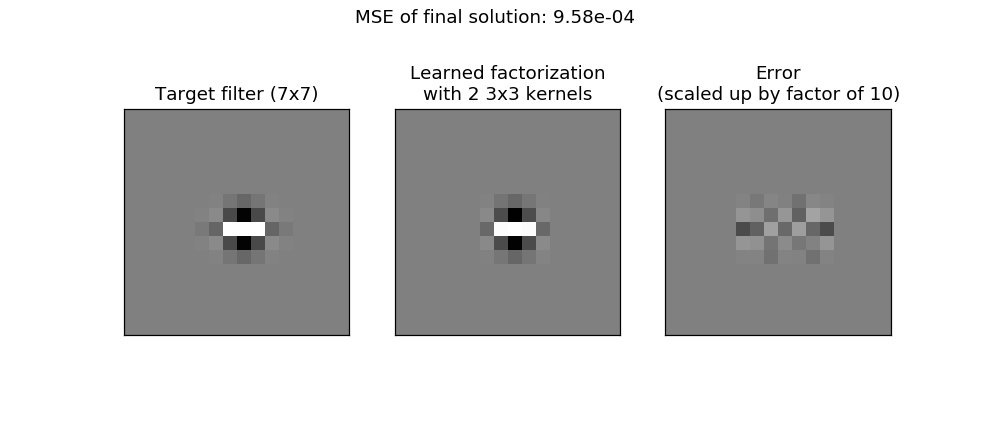# Run with different initial conditions¶

This is good, but we want to run it with different random starts and find the best over these

In :

def get_loss(x, y, net, λ, λ1, λ2):
y_out = net(x)

lmse = λ*torch.mean((y - y_out)**2)
lreg = λ1 * net.reg_loss()
l = lmse + lreg + ladmiss
return l

class MyNet(torch.nn.Module):
def __init__(self, k=3, n=2):
super().__init__()
self.w = torch.nn.ParameterList([
torch.nn.Parameter(torch.randn(1,1,k,k)) for _ in range(n)])
self.n = n
self.k = k

def reg_loss(self):
loss = torch.tensor(0., dtype=torch.float)
for i in range(self.n):
loss += torch.mean(self.w[i]**2)
return loss

def accept_weights(self, ws):
self.w = torch.nn.ParameterList([
torch.nn.Parameter(
for w in ws
])
self.n = len(ws)
self.k = ws.shape[-1]

def forward(self, X):
for i in range(self.n):
return X

def find_ws(x, target, k=3, n=2, runs=50, iters=50, λ=5000, λ1=0.1, λ2=1, lr=5e-3):
""" Matches a target filter by convolving n smaller filters

Args:
x: the impulse input
target: target filter
k: kernel size of smaller filter
n: number of successive smaller filters to use
runs: number of random starts
iters: number of steps per random start
λ: MSE lagrange multiplier
λ1: Regularization lagrange multiplier
"""
if np.abs(target.sum()) > 1e-6 and λ2 > 0:
print('Warning: the admissibility gain is non zero but the target is '

bestw = [np.zeros((1,1,k,k), dtype='float32') for _ in range(n)]

y = torch.tensor(target, dtype=torch.float32, device=dev)
if len(y.shape) == 2:
y = torch.unsqueeze(torch.unsqueeze(y, dim=0), dim=0)
# Make the target 0 mean and unit std
y = (y-y.mean())/y.std()

x = torch.tensor(x, dtype=torch.float32, device=dev)
if len(x.shape) == 2:
x = torch.unsqueeze(torch.unsqueeze(x, dim=0), dim=0)
lbest = np.inf

for N in range(runs):
try:
net = MyNet(k, n)
optim = [torch.optim.SGD([net.w[i]], lr=lr, momentum=0) for i in range(n)]

lbest_run = np.inf
bestw_run = [np.zeros((1,1,k,k), dtype='float32') for _ in range(n)]

for i in range(iters):
for j in range(n):

l = get_loss(x, y, net, λ, λ1, λ2)
l.backward(retain_graph=True)
optim[j].step()

#if torch.isnan(net.w[j]).sum() > 0:
#    raise RuntimeError
if l.item() < lbest_run:
for p in range(n):
bestw_run[p][:] = net.w[p].detach().data.cpu().numpy()[:]
lbest_run = l.item()

if lbest_run < lbest:
for p in range(n):
bestw[p][:] = bestw_run[p][:]
lbest = lbest_run
except RuntimeError:
# Nan error raised
print('Nan was raised in iter {}. Continuing...'.format(N))
continue

return lbest, bestw

In :

impulse16 = torch.zeros((1,1,16,16))
impulse16[0,0,8,8] = 1
lbest, bestw = find_ws(x=impulse16,
target=xb,
k=3,
n=2,
iters=100,
λ=100, λ1=0.1, λ2=1, lr=5e-2)

In :

# Get the output by passing impulse to network
net = MyNet()
net.accept_weights(bestw)
y_hat = net(impulse16).detach().cpu().numpy()[0,0]
y_target = torch.tensor(xb, dtype=torch.float32, device=dev)
y_target = ((y_target-y_target.mean())/y_target.std()).cpu().numpy()

# Plot
fig, ax = plt.subplots(1,3, figsize=(9,4),
subplot_kw={'xticks':[], 'yticks':[]})
ax.imshow(y_target, vmin=-5, vmax=5)
ax.imshow(y_hat, vmin=-5, vmax=5)
ax.imshow(y_target-y_hat, vmin=-.5, vmax=0.5)
fig.suptitle('MSE of final solution: {:.2e}'.format(
np.mean((y_target - y_hat)**2)));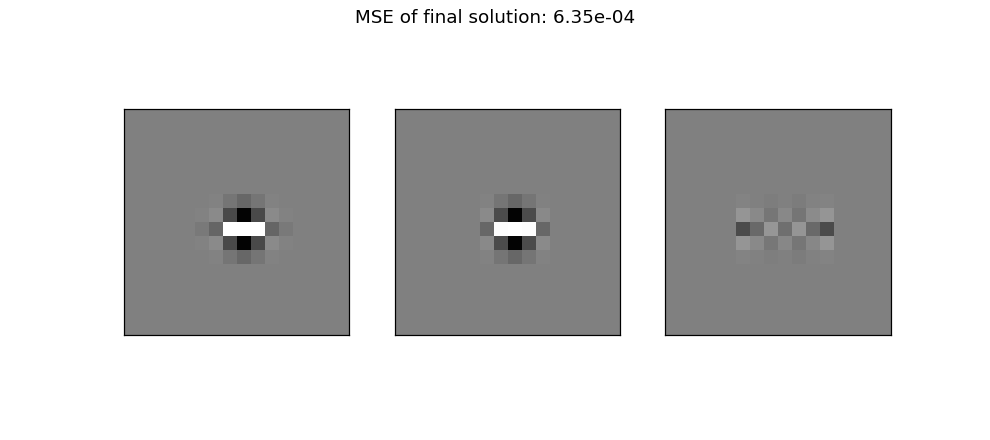# Compare to parameterization as 1 5x5¶

We know this should be pretty much perfect, so how does the above compare to a 5x5?

In :

lbest, bestw_1_5x5_lh = find_ws(impulse16, xb, k=5, n=1, iters=100)

# Get the output by passing impulse to network
x = torch.zeros(1,1,16,16)
x[0,0,8,8] = 1
for w in bestw_1_5x5_lh:
y_hat = x.detach().cpu().numpy()[0,0]

fig, ax = plt.subplots(1,3, figsize=(9,4),
subplot_kw={'xticks':[], 'yticks':[]})
ax.imshow(y_target, vmin=-5, vmax=5)
ax.imshow(y_hat, vmin=-5, vmax=5)
ax.imshow(y_target-y_hat, vmin=-.5, vmax=0.5)

fig.suptitle('MSE of final solution: {:.2e}'.format(
np.mean((y_target - y_hat)**2)));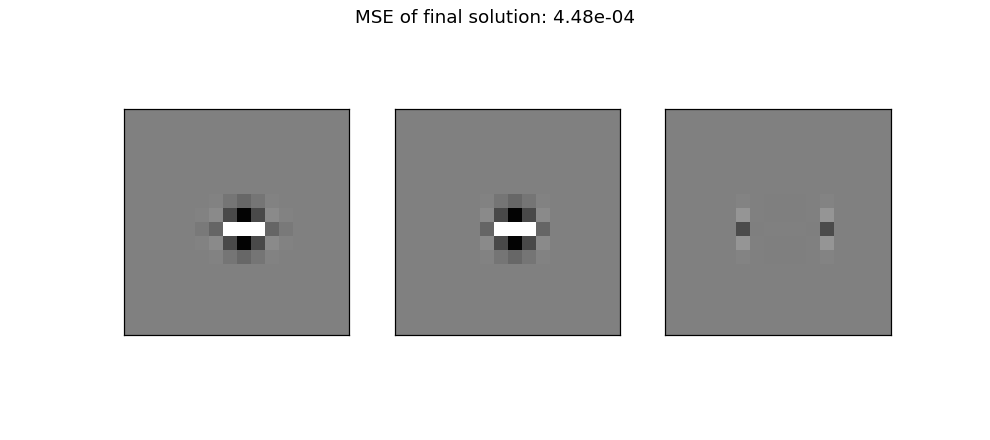# Compare to 3 3x3 kernels¶

In :

lbest, bestw_3_3x3_lh = find_ws(impulse16, xb, k=3, n=3, iters=100, λ=500)

# Get the output by passing impulse to network
net = MyNet()
net.accept_weights(bestw_3_3x3_lh)
y_hat = net(impulse16).detach().cpu().numpy()[0,0]
y_target = torch.tensor(xb, dtype=torch.float32, device=dev)
y_target = ((y_target-y_target.mean())/y_target.std()).cpu().numpy()

# Plot
fig, ax = plt.subplots(1,3, figsize=(9,4),
subplot_kw={'xticks':[], 'yticks':[]})
ax.imshow(y_target, vmin=-5, vmax=5)
ax.imshow(y_hat, vmin=-5, vmax=5)
ax.imshow(y_target-y_hat, vmin=-.5, vmax=0.5)
fig.suptitle('MSE of final solution: {:.2e}'.format(
np.mean((y_target - y_hat)**2)));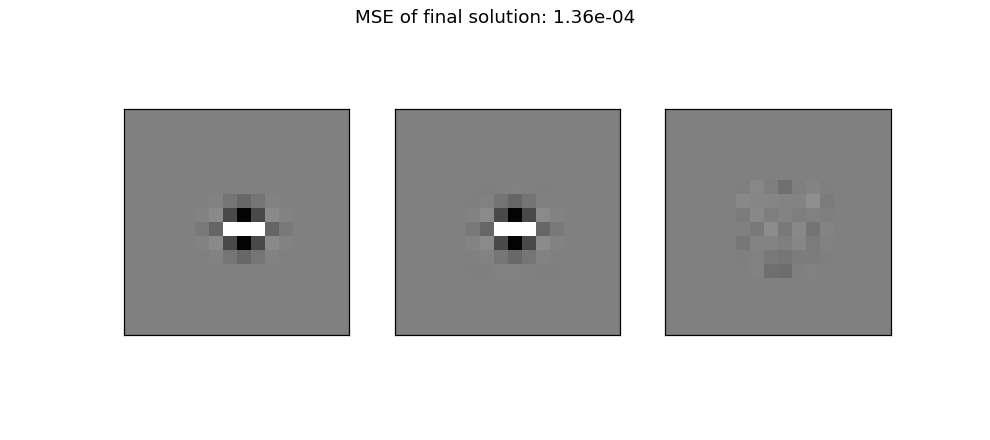# Try a different filter¶

For finality, let us also try the ll filter. Again we’ll go back to the design with 2 3x3 filters instead of 1 5x5 or 3 3x3’s

In :

lbest, bestw_2_3x3_ll = find_ws(impulse16, xb, k=3, n=2, iters=100, λ=1000, λ2=0)

# Get the output by passing impulse to network
net = MyNet()
net.accept_weights(bestw_2_3x3_ll)
y_hat = net(impulse16).detach().cpu().numpy()[0,0]
y_target = torch.tensor(xb, dtype=torch.float32, device=dev)
y_target = ((y_target-y_target.mean())/y_target.std()).cpu().numpy()

# Plot
fig, ax = plt.subplots(1,3, figsize=(9,4),
subplot_kw={'xticks':[], 'yticks':[]})
ax.imshow(y_target, vmin=-5, vmax=5)
ax.imshow(y_hat, vmin=-5, vmax=5)
ax.imshow(y_target-y_hat, vmin=-.5, vmax=0.5)
fig.suptitle('MSE of final solution: {:.2e}'.format(
np.mean((y_target - y_hat)**2)));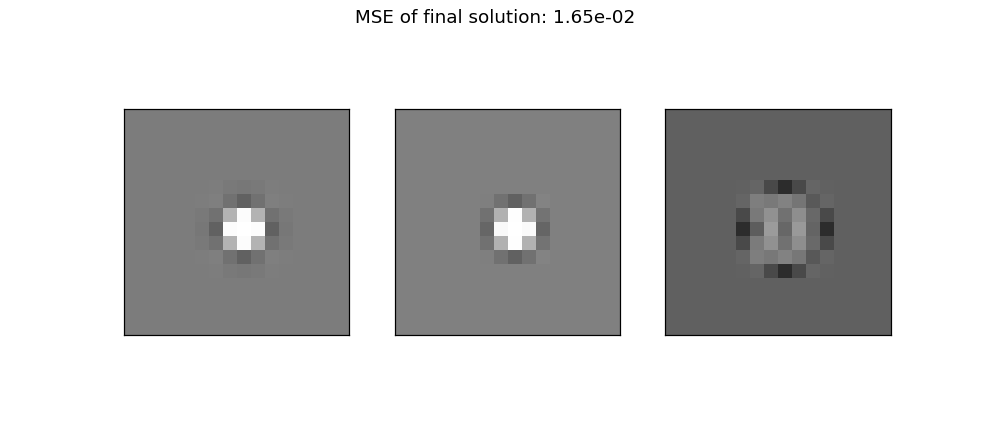# Conclusion¶

We have shown how it is possible to factorize some larger filters as convolutions of smaller filters. Note that we haven’t discussed a lot of the hyperparameter search done for the different weight factors between MSE loss, l2 loss and admissibility as well as the learning rate. These are not necessarily easy to find, and some optimization may need to be done if repeating on your own filters.